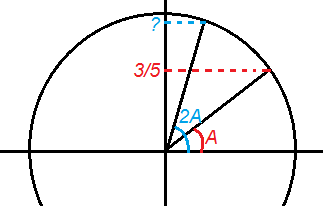# If sin(A) = 3/5, what is sin(2A)?

1 teachers like this lesson
Print Lesson

## Objective

SWBAT derive and apply the double angle and power reducing formulas.

#### Big Idea

How do the double angle formulas follow from the sum and difference formulas?

## Launch and Explore

15 minutes

The relationship between sin(A) and sin(2A) will be the driving force behind today's lesson - I want students to think about this relationship and how it relates to the sum and difference formulas that we used yesterday.

I will give students the task worksheet and have them work on the first page with their table. Students may not automatically see a connection to the work we did yesterday with the sum and difference formulas and that is okay. For now, at the very least, I want them to make sense of the problem and understand what we are trying to find.

There will probably be three main avenues that students will take to work on the first problem on the worksheet – how to find sin(2A) if sin(A) = 3/5.

1. Students who use the inverse sine on their calculator to find A, then double the angle measure and find the sine: I plan to keep an eye out for students who round the value of A. If they do, I'll make a comment that their answer will not be exactly correct. Then, I'll ask them to find another way to find this value without using a graphing calculator. The sine of 2A has to be related to the sine of A, right?

2. Students who use the sum formula from yesterday to find sin(A + A): If I see students do this, I'll encourage them to see if they could find this another way. What if they did not know this formula? Would there still be a way to find sin(2A)?

3. A student who is completely stuck and has no idea what to do: Well, I'll start by asking him/her to explain what we are looking for. Then, I'll guide them to understand that there is some angle A whose sine is 3/5, and we want to find the sine of double that angle. Finally, I will ask him/her how they could find out what angle measure A has to be. Usually, that's enough.

## Share

15 minutes

I would start by drawing a diagram of this situation (like shown below).Next I will encourage students to draw some angle A where the sine would be approximately 3/5. In front of the class, I will have a student estimate where the terminal side of the angle should be located. Then, I will choose another to draw in (2A), estimating where that should be. Then, I'll ask all of the students to estimate the sine to be based on the diagram. It is important to relate this argument back to the unit circle to give a concrete reference for students.

Now, I will choose a student who can solve this solely on their calculator to present their findings. This is a good time to talk about Question #2. Why do we need to know that angle A is in quadrant I. If necessary, I will ask students what would be different if A were in quadrant II (more on that in the video below).

Finally, I will choose a student who used sin(A + A) to share their work. After they present, I will take the time to derive the new formula sin(2A) = 2sin(A)cos(A) to make the argument clear and to give students time to reflect on their own work.

## Extend

20 minutes

After deriving the sin(2A) formula, I'll give students a few minutes to derive cos(2A) and tan(2A) in a similar way. It is important to explain what exactly it means to “derive a formula.” We, as math teachers, know exactly what that implies, and we may take it for granted that our students do as well. For this reason, I make sure that students see all three versions of cos(2A).

For Question #4 from the task worksheet, students are asked to investigate how they could write y = sin4x in their calculator if the calculator was broken and they could not type in any exponents. This has implications in calculus where writing a function without exponents simplifies the process of finding the derivative. For this reason, I direct my students to the fact that you can rewrite the function as y = (sin2x)( sin2x). Then, I encourage them to work together to see if they can use the double angle formulas to find something that is equivalent to sin2x, but that does not contain exponents.

Finally, here is an assignment to sum (no pun intended) up the last two days of sum and difference and double angle formulas.